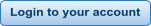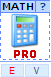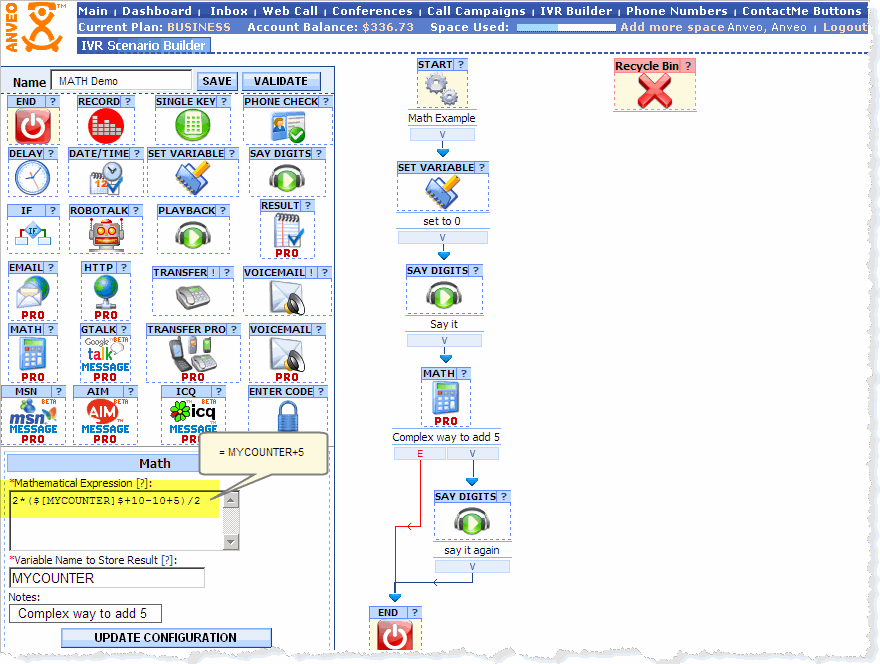Now with SMS ! Newor create new accountAdvanced Call Flow - Mathematical Operations

Math Control - perform mathematical operations with Call Flow Variables

It is possible to perform simple or complex Mathematical operations with Call Flow Variables using Math Call Control.
 Math Call Control in combination with Conditional Flow Call Control can be used to configure conditional loops.
Example
 Here is a simple Call Flow where we set MYCOUNTER Call Flow variable to 0, then Anveo will say it ( zero ) and then we use Math control to add 5 to MYCOUNTER variable and store the result back in MYCOUNTER Call Flow Variable. Finally Anveo will say 5 and hangup the call. To show what MATH control can do, please note how we add 5.